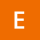Home
IT Knowledge
Inspiration
Languages
EN

# Java 8 - find first element in Stream

0 points
Created by:Evie-Grace-Noble
351

In this article, we would like to show you how to find the first element in Stream in Java.

Stream `findFirst()` returns an Optional describing the first element of this stream, or an empty Optional if the stream is empty. If the stream has no encounter order, then any element may be returned.

## Syntax

``Optional<T> findFirst()``

Where:

• `Optional` - container object which may or may not contain a non-null value.
• If a value is present, `isPresent()` will return `true` and `get()` will return the value.
• `T` - the type of objects.

## Practical example

In this example, we use the `findFirst()` function on Stream of Strings.

``````import java.util.Arrays;
import java.util.List;
import java.util.Optional;

public class Example {

public static void main(String[] args) {
// Create List of Strings
List<String> numbers = Arrays.asList("A", "B", "C", "D");

// Use Stream findFirst()
Optional<String> result = numbers.stream().findFirst();

// If the stream is empty returns empty Optional
if (result.isPresent()) {
System.out.println(result.get());
} else {
System.out.println("empty");
}
}
}``````

Output:

``A``